Exercises

# Geometry 5

Geometry in third grade introduces kids to the idea that shapes have different categories (rectangles, rhombuses, etc..) attributes (four-sided, etc...) and areas of different values. This lesson, designed by our curriculum experts, provides the guided instruction and practice that third graders need to conceptualize shapes in a deeper way. For more practice, download and print the third grade geometry worksheets recommended as part of this lesson.
This lesson includes 5 printable learning activities.

## Seashell Fractions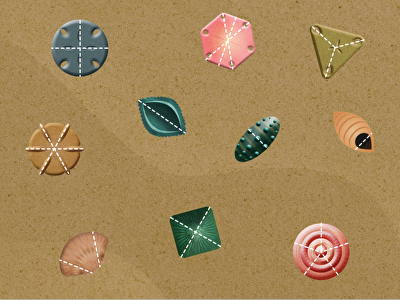Let's take this math lesson to the beach! In this fractions exercise, kids will identify shells that are split into equal parts, and answer questions about what types of fractions they see. There are a mix of seashells that show equal shares and unequal shares. This is a good visual to help your student understand that fractions are equal parts of a whole.

## 2D Shapes and Fractions with Equal Areas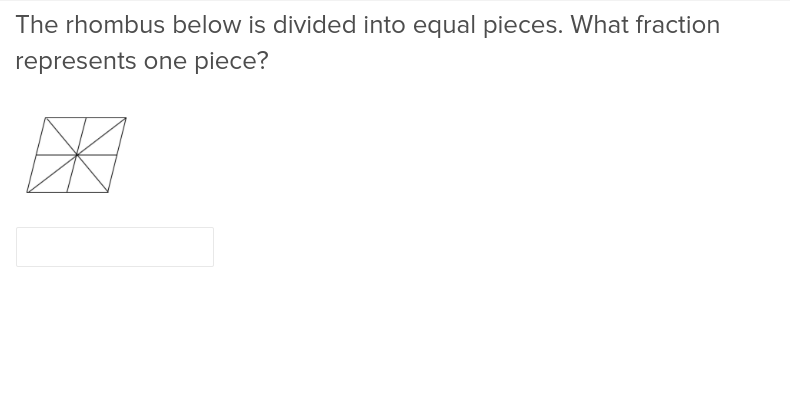Calculating the area of 2d shapes with equal fractions is simplified with this easy to understand exercise.

## 2D Shapes with Shared Attributes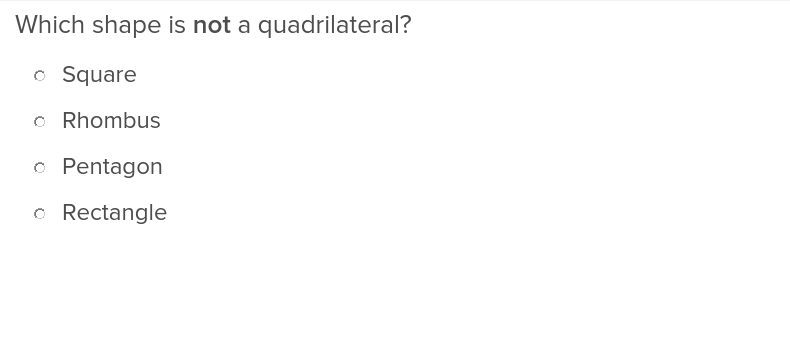This exercise will deepen students’ understanding of 2d shapes by exploring examples with shared attributes.

## Alfalfa's Out of the Box: Perimeter, Area, and Addition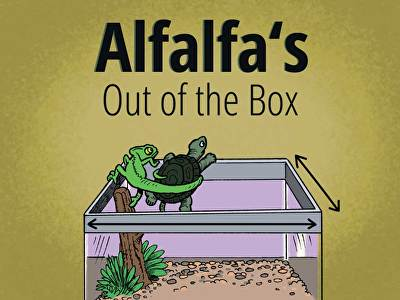Help Alfalfa plan her great escape in this interactive math story that requires the most basic of escape artist skills: math! In this exciting story full of twist, turns, and friendship, third graders can calculate area to help these two reptiles flee their confines by figuring out the area and perimeter of a rectangle. Also recommended for fourth grade, this choose-your-own-answer adventure is a blast for any species.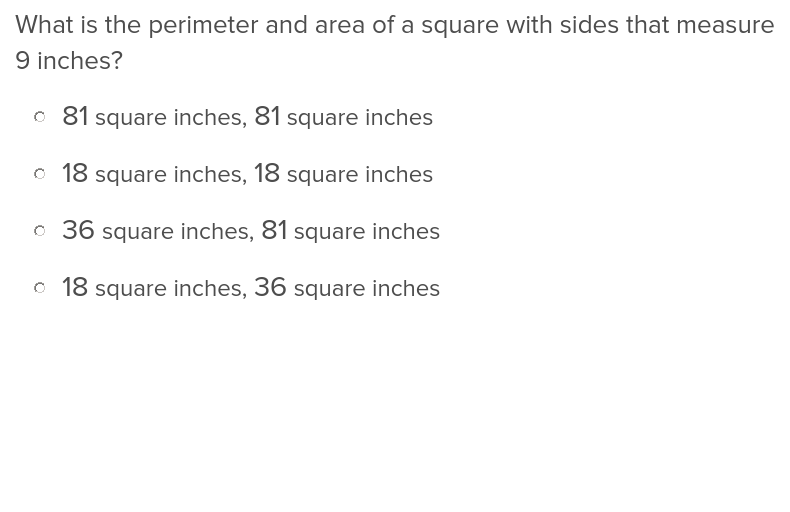This addition exercise will help students add it all up when calculating the perimeter of an object.

## City Building: Square Unit Practice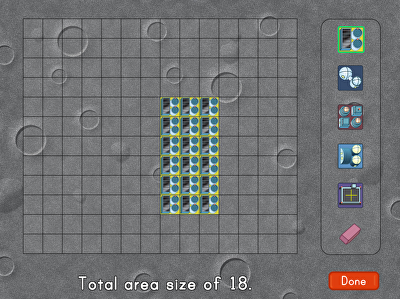Calling all space architects! You have landed on the moon, and now you need to start building a colony for future inhabitants. In this game, kids build structures for their moon colony according the given area as they build key geometry skills around area in square units. Great for third grade math students, City Building: Square Unit Practice introduces the concept of area in an engaging, interactive way.

## Area of a Rectangle Using Square Units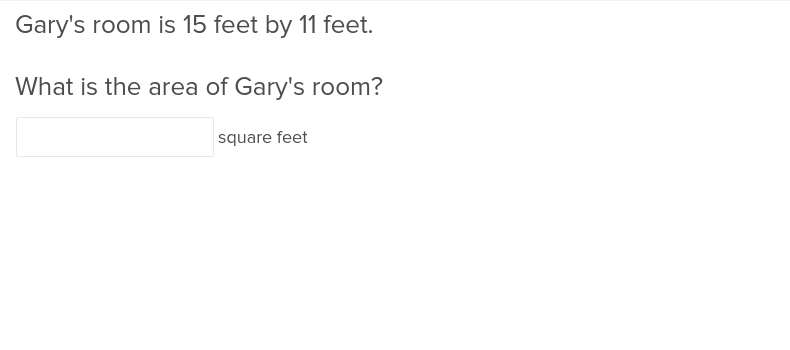This exercise will students how to calculate the area of a rectangle with ease using square units.